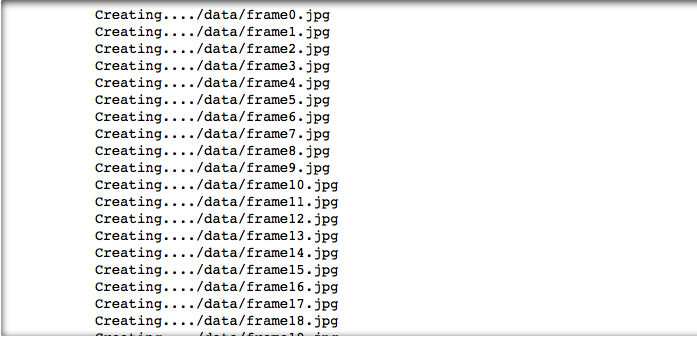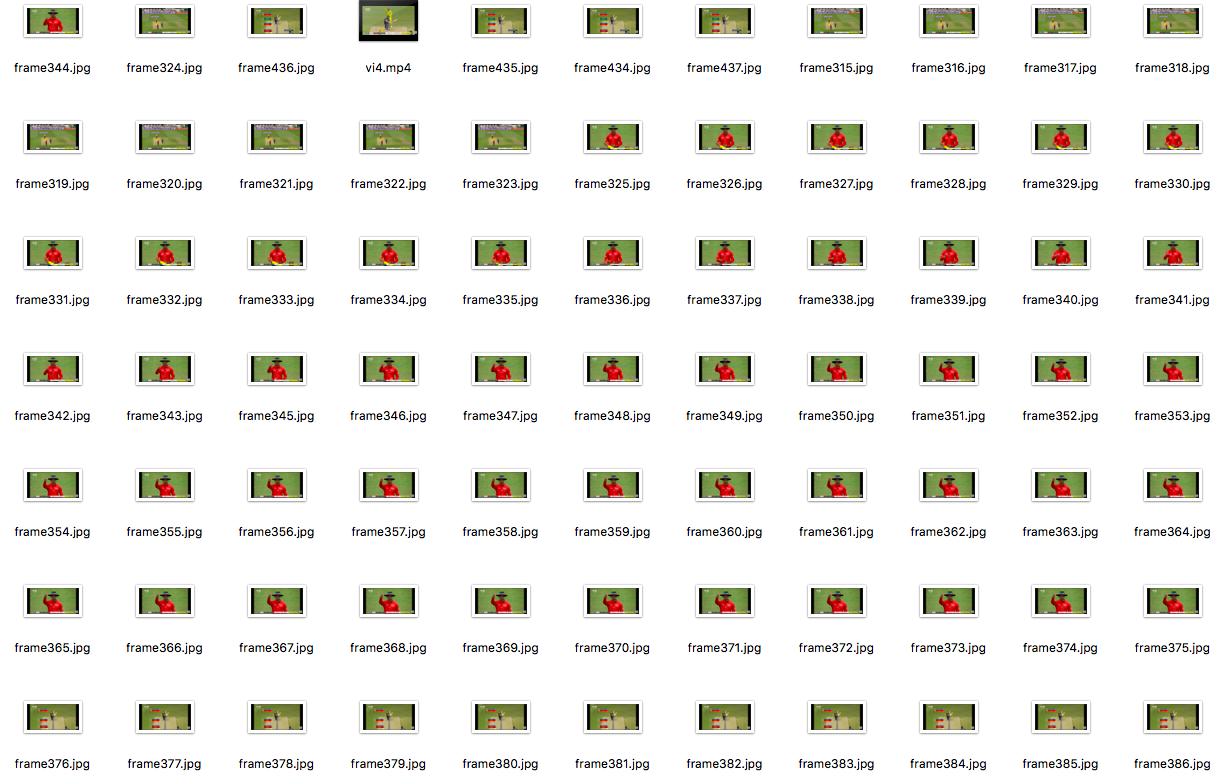GFG App
Open AppBrowser
Continue

# Extract images from video in Python

OpenCV comes with many powerful video editing functions. In current scenario, techniques such as image scanning, face recognition can be accomplished using OpenCV.

Image Analysis is a very common field in the area of Computer Vision. It is the extraction of meaningful information from videos or images. OpenCv library can be used to perform multiple operations on videos.

Modules Needed:

```import cv2
import os
```

Function Used :

`VideoCapture(File_path) : Read the video(.mp4 format)`

``` read() : Read data depending upon the type of object that calls ```

`imwrite(filename, img[, params]) : Saves an image to a specified file.`

Below is the implementation:

 `# Importing all necessary libraries ` `import` `cv2 ` `import` `os ` ` `  `# Read the video from specified path ` `cam ``=` `cv2.VideoCapture(``"C:\\Users\\Admin\\PycharmProjects\\project_1\\openCV.mp4"``) ` ` `  `try``: ` `     `  `    ``# creating a folder named data ` `    ``if` `not` `os.path.exists(``'data'``): ` `        ``os.makedirs(``'data'``) ` ` `  `# if not created then raise error ` `except` `OSError: ` `    ``print` `(``'Error: Creating directory of data'``) ` ` `  `# frame ` `currentframe ``=` `0` ` `  `while``(``True``): ` `     `  `    ``# reading from frame ` `    ``ret,frame ``=` `cam.read() ` ` `  `    ``if` `ret: ` `        ``# if video is still left continue creating images ` `        ``name ``=` `'./data/frame'` `+` `str``(currentframe) ``+` `'.jpg'` `        ``print` `(``'Creating...'` `+` `name) ` ` `  `        ``# writing the extracted images ` `        ``cv2.imwrite(name, frame) ` ` `  `        ``# increasing counter so that it will ` `        ``# show how many frames are created ` `        ``currentframe ``+``=` `1` `    ``else``: ` `        ``break` ` `  `# Release all space and windows once done ` `cam.release() ` `cv2.destroyAllWindows() `

Output:All the extracted images will be saved in a folder named “data” on the system.My Personal Notes arrow_drop_up